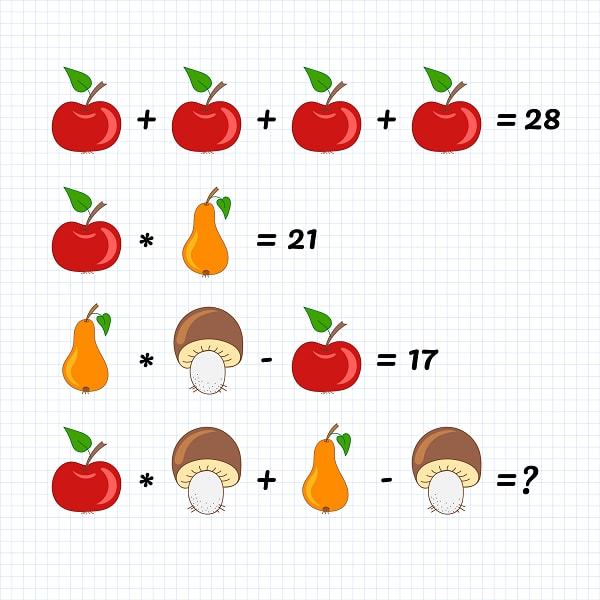August 10, 2022

Read Latest News here at Blogs Tab

# Engaging and Challenging Math puzzlesLast Updated on March 22, 2022 by admin

It can sound a bit harsh but many of the students find the subject of mathematics very tough and boring. To bring about the engagement and interest of students in math, activities like math puzzles and math games are one of the best things. Math puzzles and maths games are some of the oldest methods that challenge students and enhance the problem-solving and tactical thinking of students. Math puzzles form an integral part of recreational mathematics which consists of a specific set of rules and methods to solve them. In this article, we will learn about the various types of math puzzles that you can play to enhance your interest in mathematics.

## Study Math Online from the Best Teachers at Cuemath

Mathematics is a very different subject as compared to others. Students need conceptual clarity along with constant practice in this subject. Due to the ongoing pandemic, things have drastically changed and the whole education system has come online. Although there are many options available online, students prefer to study math online from Cuemath as they have the best teachers of the subject who focus extensively on the foundational concepts of the subject so that students do not face any problems in the complex topics. For regular practice, recreational activities like puzzles, games, worksheets, and so on are conducted periodically. If you want to study math online from Cuemath, visit their website and book a free session to experience their way of teaching.

## Various Types of Engaging and Challenging Math Puzzles

There are innumerable math puzzles that students play across the globe. These math puzzles help them to increase their interest in math along with enhancing their tactical, analytical, and thinking capabilities. Here is the list of some of the most interesting and engaging math puzzles.

• Math Crossword Puzzles: You must have come across crosswords in daily newspapers. In daily newspapers, we usually have alphabetical crosswords. We can take a crossword and make it math to prepare the math crossword puzzles. It is a highly addictive and adaptable game that you can play to enhance your problem-solving techniques. If played regularly, it will help you master the arithmetic operations which are addition, multiplication, division, and subtraction.
• Math Problem Search: A word-search style math puzzle can be created by teachers to help students. Master arithmetic operations like addition, division, subtraction, and multiplication. In this math puzzle, students have to search for hidden math equations and solve them accordingly.
• 2048: It is a math puzzle that you will have to play on your mobile phones. 2048 is a single-player game that requires you to slide tiles. That consist of numbers to make them bigger numbers and eventually,. You have to reach the number 2048. It is a highly addictive math puzzle game and not as easy as it sounds. Students need to think strategically before proceeding with any few moves. 2048 is a great math puzzle that can help students learn the concept of exponents effectively.
• Magic Square: This math puzzle has been around for thousands of years. It was created by Arabs and later on was introduced to Western civilization during the period of the Renaissance. Magic squares can have a variety of grids, however, the most popular and the most accessible magic square is the square that consists of three by three grids. As compared to other games, it is a tough puzzle and requires students to think tactically. In this math puzzle, students need to arrange numbers starting from 1 to 9 in a way that the sum of any three straight lines (vertically, horizontally, and diagonally) is 15.

Various other math puzzles that you can look out for are sudoku, perimeter magic triangle, flexagon, turn the fish, join the dots, and so on.